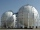# Spherical segment

Spherical segment with height h=6 has a volume V=134. Calculate the radius of the sphere of which is cut this segment.

Result

r =  3.18

#### Solution:Leave us a comment of example and its solution (i.e. if it is still somewhat unclear...):

Showing 1 comment:Math student
Can you show the step by step process#### To solve this example are needed these knowledge from mathematics:

Tip: Our volume units converter will help you with converion of volume units.

## Next similar examples:A domed stadium is in the shape of spherical segment with a base radius of 150 m. The dome must contain a volume of 3500000 m³. Determine the height of the dome at its centre to the nearest tenth of a meter.
2. Hemispherical hollowThe vessel hemispherical hollow is filled with water to a height of 10 cm =. How many liters of water are inside if the inside diameter of the hollow is d = 28cm?
3. Spherical capFrom the sphere of radius 18 was truncated spherical cap. Its height is 12. What part of the volume is spherical cap from whole sphere?
4. Spherical tankThe tank of a water tower is a sphere of radius 35ft. If the tank is filled to one quarter of full, what is the height of the water?
5. TV transmitterThe volume of water in the rectangular swimming pool is 6998.4 hectoliters. The promotional leaflet states that if we wanted all the pool water to flow into a regular quadrangle with a base edge equal to the average depth of the pool, the prism would have.
6. Axial sectionAxial section of the cone is an equilateral triangle with area 208 dm2. Calculate the volume of the cone.
7. CuboidCuboid with edge a=16 cm and body diagonal u=45 cm has volume V=11840 cm3. Calculate the length of the other edges.
8. Cone A2VSurface of cone in the plane is a circular arc with central angle of 126° and area 415 cm2. Calculate the volume of a cone.
9. Cube in a sphereThe cube is inscribed in a sphere with volume 6116 cm3. Determine the length of the edges of a cube.
10. Transforming cuboidCuboid with dimensions 8 cm, 13, and 16 cm is converted into a cube with the same volume. What is its edge length?
11. Lateral surface areaThe ratio of the area of the base of the rotary cone to its lateral surface area is 3: 5. Calculate the surface and volume of the cone, if its height v = 4 cm.
12. Ratio of volumesIf the heights of two cylindrical drums are in the ratio 7:8 and their base radii are in the ratio 4:3. What is the ratio of their volumes?
13. CubesOne cube is inscribed sphere and the other one described. Calculate difference of volumes of cubes, if the difference of surfaces in 257 mm2.
14. Sphere VSFind the surface and volume of a sphere that has a radius of 2 dm.The radius of the sphere we reduce by 1/3 of the original radius. How much percent does the volume and surface of the sphere change?The rectangular cuboid has a surface area 5334 cm2, its dimensions are in the ratio 2:4:5. Find the volume of this rectangular cuboid.The swimming pool is 10 m wide and 8 m long and 153 cm deep. How many hectoliters of water is in it, if the water is 30 cm below its upper edge?